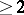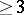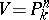# Birational geometry

(diff) ← Older revision | Latest revision (diff) | Newer revision → (diff)

The branch of algebraic geometry in which the main problem is the classification of algebraic varieties up to birational equivalence (cf. Birational mapping). Over a fixed field of constantsevery class of birationally equivalent varieties defines a finitely generated field over, which is isomorphic to the field of rational functions on any variety in this class. Conversely, to each such field corresponds a class of birationally equivalent varieties — the model(s) of this field. Thus, the birational classification of algebraic varieties is equivalent to the classification (up to a-isomorphism) of the finitely generated fields regular over.

The most general birational invariant is the dimension of an algebraic variety. For one-dimensional algebraic varieties — irreducible algebraic curves — each class of birational equivalence contains a non-singular model — a smooth projective curve, which is unique up to-isomorphisms. Accordingly, the birational classification of algebraic curves is reduced to the classification, up to-isomorphisms, of smooth projective curves, which leads to the moduli problem. For dimensionsthe problem becomes much more complicated. The very existence of a smooth model constitutes a problem of resolution of singularities of algebraic varieties, which at the time of writing (1986) has been positively solved only for surfaces and for varieties of arbitrary dimension over a field of characteristic zero. In such a case, if such models exist at all, there are infinitely many of them in the class of birationally equivalent varieties. The minimal models (cf. Minimal model) take a special place among such models. Their birational classification is often identical with classification up to a-isomorphism, as in the case of curves. However, this is not true in the general case, even for (both rational and ruled) surfaces.

The principal results in the classification of algebraic surfaces were obtained by geometers of the Italian school . Only isolated results are so far (1986) available , ,  for varieties of dimensions of.

The principal discrete birational invariants of smooth complete algebraic varieties over a fieldof characteristic zero include the arithmetic genus, the geometric genus, the multiple genus, the dimension of the space of regular differential forms, the Severi torsion, the fundamental group and the Brauer group.

One of the most important problems in birational geometry is the problem of rationality of algebraic varieties, i.e. the problem of the description of rational varieties — varieties which are birationally equivalent to a projective space, cf. Rational variety.

If the field of constants is algebraically non-closed, the problems in birational geometry are closely connected with the arithmetic of algebraic varieties (cf. Algebraic varieties, arithmetic of). The important problem in such a case is that of describing the birational-forms on a given varietyover a field, in particular if, say,is a projective space over. An important part of this problem is the description of the group of birational transformations of the variety.

How to Cite This Entry:
Birational geometry. Encyclopedia of Mathematics. URL: http://encyclopediaofmath.org/index.php?title=Birational_geometry&oldid=12684
This article was adapted from an original article by I.V. DolgachevV.A. Iskovskikh (originator), which appeared in Encyclopedia of Mathematics - ISBN 1402006098. See original article